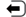Latest web development tutorials

# VBScript UBound functionComplete VBScript Reference

UBound function returns the maximum dimension of indication array index.

Tip: UBound function and the function LBound used together, can determine the size of the array.

### grammar

UBound(arrayname[,dimension])

arrayname 必需。数组变量的名称。
dimension 可选。要返回哪一维的下界。 1 = 第一维， 2 = 第二维，以此类推。默认是 1 。

## Example 1

<script type="text/vbscript">

days=Array("Sun","Mon","Tue","Wed","Thu","Fri","Sat")
document.write(LBound(days) & "<br />")
document.write(UBound(days) & "<br />")

</script>

Examples of the above output:

0
6

try it"

## Example 2

A two-dimensional array:

<script type="text/vbscript">

Dim food(2,3)
food(0,0)="Apple"
food(0,1)="Banana"
food(0,2)="Orange"
food(0,3)="Lemon"
food(1,0)="Pizza"
food(1,1)="Hamburger"
food(1,2)="Spaghetti"
food(1,3)="Meatloaf"
food(2,0)="Cake"
food(2,2)="Icecream"
food(2,3)="Chocolate"
document.write(LBound(food,1) & "<br />")
document.write(UBound(food,1) & "<br />")
document.write(LBound(food,2) & "<br />")
document.write(UBound(food,2) & "<br />")

</script>

Examples of the above output:

0
2
0
3

try it"Complete VBScript Reference